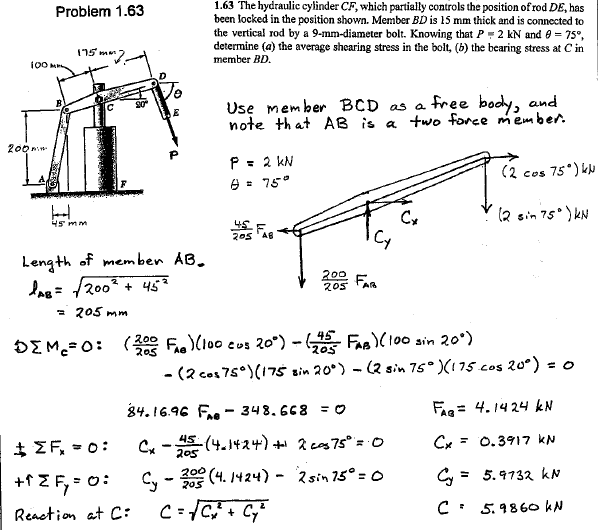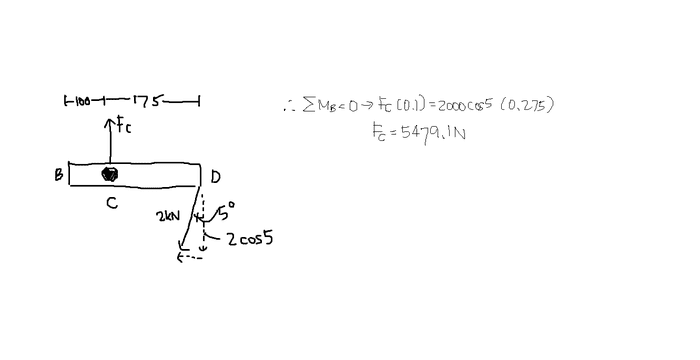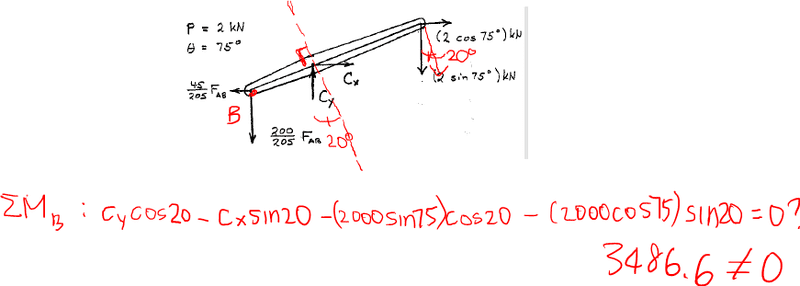## Homework Statement

Here is the question along with part of the solution. I am online concerned about finding the force at C.## The Attempt at a SolutionFor my solution I decided I would sum the moments about point B. However I ended up getting the wrong answer for the force at C. Does anyone know why?

PhanthomJay
Homework Helper
Gold Member
You didn't follow the solution manual. You should first check your geometry to see that the angle you denote as 5 degrees is actually 15 degrees. But then if you sum moments about B, C has both vertical and horizontal components, it is not perpendicular to the member.

You didn't follow the solution manual. You should first check your geometry to see that the angle you denote as 5 degrees is actually 15 degrees. But then if you sum moments about B, C has both vertical and horizontal components, it is not perpendicular to the member.

Ah, I reoriented BD so that the angle there is 5 degrees instead of 15 :P. So you are saying force C will not be perpendicular to BD?

I just summed the moments about B using their answers. I converted the forces C and P so that they are perpendicular to the link BD and summed the moments. The moment does not equal to zero. Is this an error?

Here is my work: